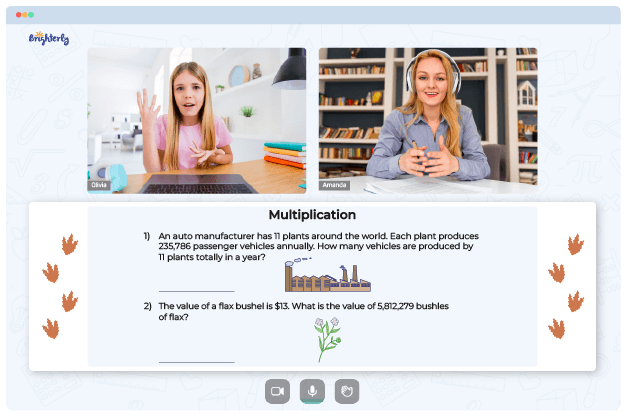# Basic Multiplication Worksheets

Multiplication is one of the fundamental mathematical operations that children are introduced to during their early years of schooling. Basic multiplication involves multiplying two numbers together to get a product. As a result, basic multiplication worksheets PDF are often used as teaching aids to ensure children have a strong foundation in multiplication.

This article will explore the purpose and benefits of using basic multiplication worksheets and provide some tips on using them effectively.

Basic multiplication worksheets are designed to help children practice and master multiplication facts, which are the fundamental multiplication equations. They typically include a series of math problems with two numbers a child must multiply to get a product. Basic multiplication worksheets grade 2 are generally tailored to different age groups and skill levels, from simple problems like 2 x 2 to advanced ones like 8 x 9.

Math for Kids

Is Your Child Struggling With Math?
1:1 Online Math Tutoring## How to Use Basic Multiplication Worksheets

Starting with easy problems is an excellent way to build your child’s confidence and prepare them for more challenging problems. For example, you can begin by giving your child fundamental multiplication problems that are easy to solve, such as 2 x 2 or 3 x 4. Once they have mastered these, they move on to more challenging problems.

Repetition is vital when it comes to basic math multiplication worksheets. Therefore, ensuring that your child practices the same multiplication fact multiple times until they have it memorized is essential. The more they practice, the more automatic the multiplication process becomes, which leads to quicker recall when kids face similar problems in the future.

Timed tests are an excellent way to help your child build speed and accuracy. For example, giving your child a set amount of time, such as 60 seconds, to solve as many multiplication basic facts worksheets problems as possible will help them learn to solve problems quickly and accurately. It will also encourage them to work efficiently under pressure.

## Benefits of Basic Multiplication Worksheets

One of the significant benefits of using basic multiplication and division worksheets is that they help children develop their math skills. Children can hone their problem-solving, logical thinking, and mental math skills by regularly practicing basic multiplication facts worksheets. In addition, they can learn to identify patterns and relationships between numbers, which leads to a better understanding of mathematical concepts.

As children become more skilled at solving basic multiplication printable worksheets problems, their confidence in their math abilities will grow. Confidence can translate to other areas of their lives and help them become more successful students. In addition, they will feel more capable of tackling challenging math problems and tasks in other academic subjects, which will finally lead to a sense of accomplishment and confidence.

Struggling with Multiplication?• Is your child finding it challenging to master multiplication fundamentals?
• An online tutor could be the solution.

Does your child struggle to understand the basics of multiplication? Try lessons with an online tutor.